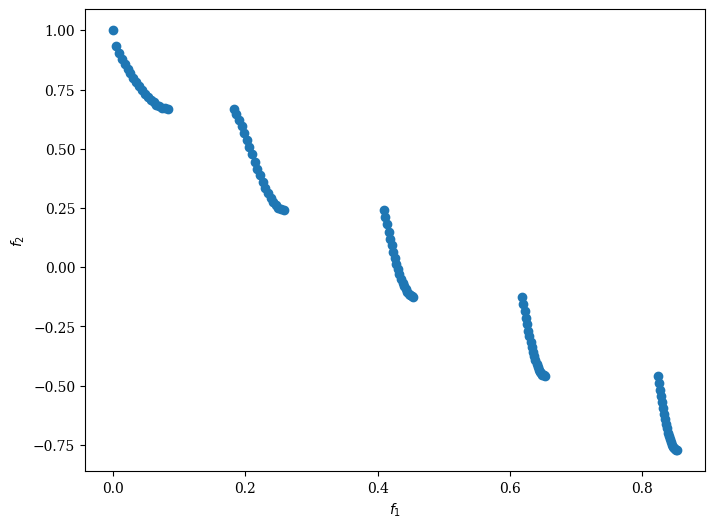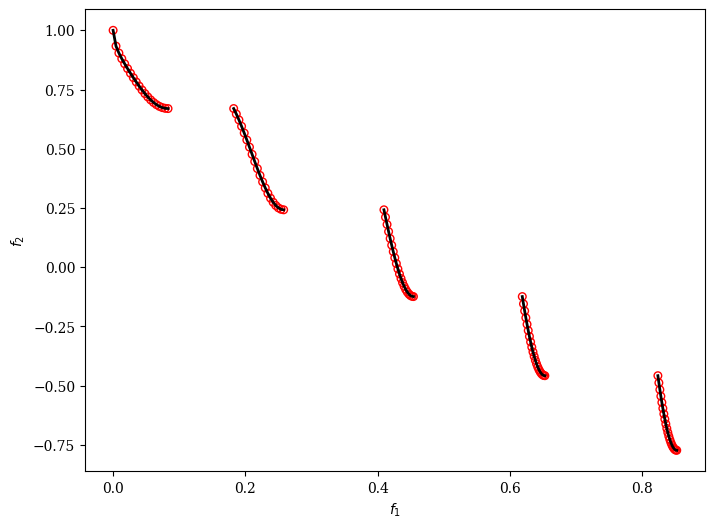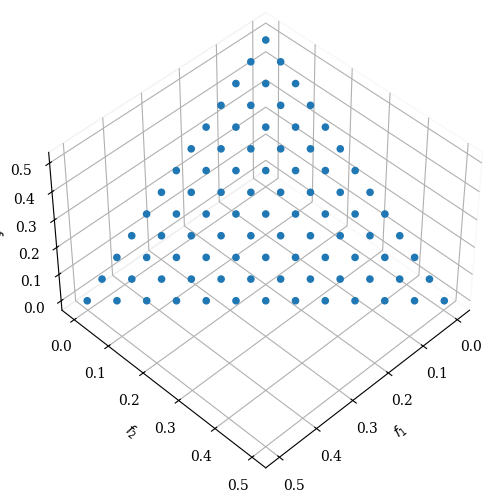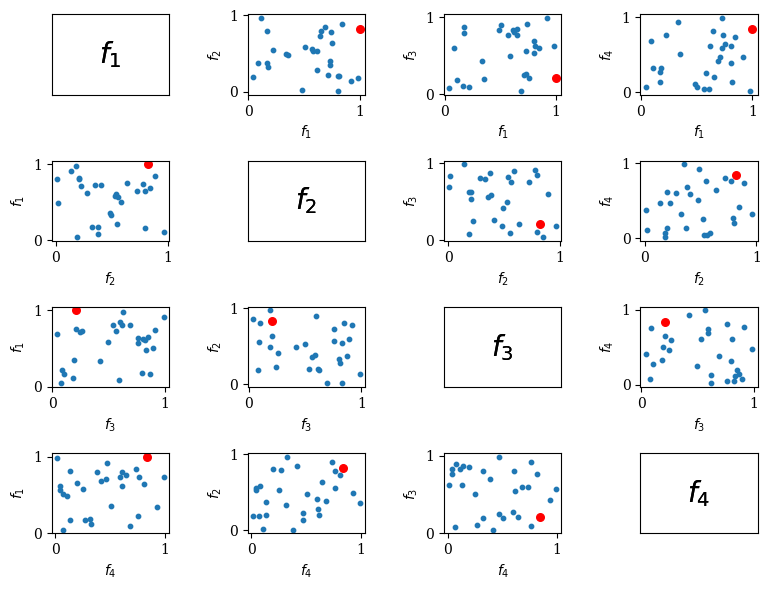Version: 0.5.0

# Scatter Plot¶

The traditional scatter plot is mostly used for lower dimensional objective spaces.

## Scatter 2D¶

:

from pymoo.visualization.scatter import Scatter
from pymoo.factory import get_problem, get_reference_directions

F = get_problem("zdt3").pareto_front()

:

<pymoo.visualization.scatter.Scatter at 0x7fded8688f70>The plot can be further customized by supplying a title, labels, and by using the plotting directives from matplotlib.
:

F = get_problem("zdt3").pareto_front(use_cache=False, flatten=False)
plot = Scatter()
plot.show()

:

<pymoo.visualization.scatter.Scatter at 0x7fded862ee20>## Scatter 3D¶

:

ref_dirs = get_reference_directions("uniform", 3, n_partitions=12)
F = get_problem("dtlz1").pareto_front(ref_dirs)

plot = Scatter()
plot.show()

:

<pymoo.visualization.scatter.Scatter at 0x7fdec6b00130>## Scatter ND / Pairwise Scatter Plots¶

:

import numpy as np
F = np.random.random((30, 4))

plot = Scatter(tight_layout=True)
plot.show()

:

<pymoo.visualization.scatter.Scatter at 0x7fdec71aa250>## API¶

class pymoo.visualization.scatter.Scatter(self, angle=(45, 45), **kwargs)

Scatter Plot

Parameters
axis_styledict

Most of the plots consists of an axis. The style of the axis, e.g. color, alpha, …, can be changed to further modify the plot appealing.

endpoint_styledict

Endpoints are drawn at each extreme point of an objective. This style can be modified.

labelsstr or list

The labels to be used for each variable provided in the plot. If a string is used, then they will be enumerated. Otherwise, a list equal to the number of variables can be provided directly.

Other Parameters
figsizetuple

The figure size. Default (figsize=(8, 6)). For some plots changing the size might have side-effects for position.

titlestr or tuple

The title of the figure. If some additional kwargs should be provided this can be achieved by providing a tuple (“name”, {“key” : val}).

legendstr

Whether a legend should be shown or not.

tight_layoutbool

Whether tight layout should be used.

cmapcolormap

For some plots different kind of colors are used. The colormap can be changed to modify the color sequence for the plots.

Methods

 plot set_labels